Journal of Integer Sequences, Vol. 3 (2000), Article 00.1.1

## Moments of Generalized Motzkin Paths

### Robert A. Sulanke Boise State University Boise, ID 83725 USA Email address: sulanke@math.idbsu.edu

Abstract: Consider lattice paths in the plane allowing the steps (1,1), (1,-1), and (w,0), for some nonnegative integer w. For n > 1, let E(n,0) denote the set of paths from (0,0) to (n,0) running strictly above the x-axis except initially and finally. Generating functions are given for sums of moments of the ordinates of the lattice points on the paths in E(n,0). In particular, recurrencess are derived for the cardinality, the sum of the first moments (essentially the area), and the sum of the second moments for paths in E(n,0). These recurrences unify known results for w= 0, 1, 2, i.e. those for the Dyck (or Catalan), Motzkin, and Schröder paths, respectively. The sum of the second moments is seen to equal the number of unrestricted paths running from (0,0) to (0,n-2).

Contents:
1. Introduction: The paths and their moments
2. The recurrences
3. Enumerating restricted paths
4. Factorial moments
5. Area and second moments
6. Relating second moments to central numbers
7. Examples
8. Related studies
9. Bibliography

1.   Introduction: the paths and their moments

Let w be a fixed nonnegative integer. We will consider those lattice paths in the Cartesian plane whose permitted step types are the up diagonal step (1,1) denoted by U, the down diagonal step (1,-1) denoted by D, and the horizontal step (w,0) denoted by H. When w= 0, only U-steps and D-steps are permitted. We weight the steps by assigning 1 to each U-step, 1 to each D-step, and an indeterminate t to each H-step. The t-weight of a path P, denoted by |P|, is the product of the weights of its steps; and the t-weight of a set of paths S, denoted by |S|, is the sum of the t-weights of the paths in S.

Often we will suppress the parameter w and the indeterminate t in our notation. Let U(x,y) denote the set of all unrestricted lattice paths using the permitted step types and running from (0,0) to (x,y). We define the set of generalized Motzkin paths, denoted by M(x,y), to be the set of paths in U(x,y) that never run below the x-axis except initially and perhaps finally. Of particular interest is the set of elevated paths, denoted by E(x,y), consisting of those paths in M(x,y) that never touch the x-axis except initially and perhaps finally. For an example, see Figure 1 and the left column of Table 1 which give the four paths in E(5,0) when w = 1.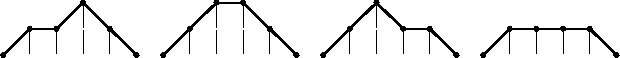Let fn(w) denote |E(n,0) | for n >= 2, with f0(w) = f1(w) = 0. For t = 1, there are three classical sequences covered by this notation, specifically for w = 0, 1 or 2. When w = 0, there are no horizontal steps and the paths of E(n,0) are the so-called elevated Dyck (or Catalan) paths; the corresponding sequence (fn(0))n >= 2 = (1, 0, 1, 0, 2, 0, 5, 0, 14, . . . ) is the sequence of (aerated) Catalan numbers. For w = 1 and t = 1, (fn(1))n >= 2 is the sequence of Motzkin numbers. For w = 2 and t = 1, (fn(2))n >= 2 is the sequence of (aerated) large Schröder numbers. See Table 2 in Section 7. In 1948, using different indexing, Motzkin  introduced the sequence (fn(1))n >= 2, where fn(1) denotes the number of ways to join n-2 points on a circle by nonintersecting chords. Donaghey and Shapiro  made an early study of this sequence which included lattice paths equivalent to those of M(n,0) with w= 1.

Consider a path P in E(n,0) as a rectilinear curve. Let (0, P(0)), (1, P(1)), ..., (n, P(n)) be the list of all lattice points (points with integer coordinates) on the path P. We will refer to the values P(0), P(1), P(2), ..., P(n) as the path ordinates of P. Define the rth moment of P to be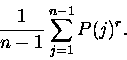The zeroth moment of P equals 1. By the elementary formula for the area of a trapezoid, we see that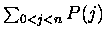equals the area bounded by the path P and the x-axis.

 path contribution contribution contribution contribution to f5(1) to g5(1) to total area to h5(1) UHUDD t 5t/4 5 7t/4 UUHDD t 6t/4 6 10t/4 UUDHD t 5t/4 5 7t/4 UHHHD t3 4t3/4 4 4t3/4

Table 1: This table gives the contributions to f5(1) = 3t+t3, g5(1) = 4t+t3, h5(1) = 6t+t3, and the total area by the the four paths of E(5,0) for w = 1.

For fixed w >= 0 and for n >= 2, we define the following sums of t-weighted moments for the path set E(n,0):

 fn(w) =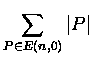(1) an(w) =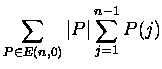(2) gn(w) =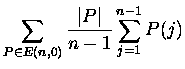(3) hn(w) =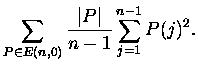(4)

The main results of this paper are the three recurrences for these sequences, given uniformly in equations (5), (6), and (7), and the generating function for the factorial moments given in Proposition 5. In Section 2 we state these recurrences, which we then establsh by generating function methods in Sections 3, 4, and 5. In Section 6 we prove a surprising result relating second moments to central unrestricted numbers. In Section 7 we will give some examples of these sequences.

2.   The recurrences

For any w >= 0, consider the following unified set of recurrences for the sequences, (fn)n >= 2, (gn)n >= 2, and (hn)n >= 2, which we have defined above in terms of t-weighted elevated paths:

 n fn = 4(n-3) fn-2 + (2n - 3 w ) t fn-w - (n - 3 w ) t2 fn-2w, (5) (n-1)gn = 4(n-3) gn-2 + (2n - 2w-2)tgn-w - (n - 2 w-1)t2 gn-2w, (6) (n-2)hn = 4(n-3) hn-2 + (2n - w - 4 )thn-w - (n - w - 2)t2 hn-2w. (7)

These recurrences are valid except for certain initial values, as specified in the propositions below. We first state these recurrences for the known case of elevated Dyck (or Catalan) paths.

Proposition 1.   For w = 0, the sequences (fn(0))n >= 2, (gn(0))n >= 2, and (hn(0))n >= 2 satisfy

 n fn(0) = 4(n-3) fn-2(0) (8) (n-1) gn(0) = 4(n-3) gn-2(0) (9) (n-2) hn(0) = 4(n-3) hn-2(0) (10)

for n >= 3, subject to the initial conditions that fn(0) = gn(0) = hn(0) = 0 for n < 2 and f2(0) = g2(0) = h2(0) = 1.

The proof of this Proposition is covered by the proofs of Propositions 2, 3, and 4. Recurrence (8) dates from about 1758, when Euler  recorded it, slightly re-indexed, when he and Segner  were considering counting triangulations of convex polygons. See Section 8. It follows immediately that, for k >= 0,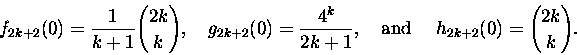(11)

For w >= 1, we have the following more general result, which in proved in the next section:

Proposition 2.   For w >= 1, the sequence (fn )n >= 2 satisfies recurrence (5) for n > 2w, with initial values satisfying fn = fn(0) + (n-w-1)t fn-w(0) for n <= 2w.

With an = an(w) denoting the t-weighted area,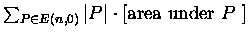, the trapezoidal area formula shows that an = (n-1)gn for all n >= 2. The following result, proved in Section 5, generalizes one of Kreweras  for w = 2 and t = 1.

Proposition 3.   For w >= 1, (gn)n >= 2 satisfies recurrence (6) for n > w + 2, with initial values gn = gn(0) for n <= w + 2, and gw+2 = gw+2(0) + t. Equivalently, for w >= 1, the sequence (an)n >= 2 satisfies the recurrence

 an = 4 an-2 + 2 tan-w - t2an-2w (12)

for n > w + 2, with initial values an = (n-1)gn(0) for n <= w + 2, and aw+2 = (w+1)gw+2(0) + (w+1)t.

We remark that (10) is a well-known recurrence for the central binomial coefficients. In the case when w = 1 with t = 1, recurrence (7) dates from 1764, as Euler  proved that the central trinomial coefficients satisfy this recurrence when appropriately re-indexed. Our knowledge that these central coefficients are solutions to the recurrences (7) and (10) led to an interesting relationship between second moments and central numbers of the form |U(n,0)| for arbitrary w. Specifically, in Section 6 we will see that |U(n-2, 0 )| satisfies a recurrence that is also satisfied by hn(t); thus we have a proof of identity (13) below. The proof of the first part of the following appears in Section 5.

Proposition 4.   For w >= 1, the sequence (hn)n >= 2 satisfies recurrence (7) for n >= 3, with the initial values hn = 0 for n < 2 and h2 = 1. Moreover, for any w and for n >= 2,

 hn = |U(n-2,0)|. (13)

3.   Enumerating restricted paths

Consider the generating function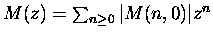. With the exception of the point path, each path in M(n,0) either begins with an H-step or immediately leaves the x-axis and then later returns for a first time. Consequently, with L denoting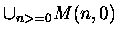and with juxtaposition indicating concatenation, we have a decomposition that defines L recursively: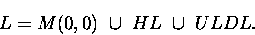With z marking a horizontal unit and with t marking each H-step, the decomposition yields

 M(z) = 1 + tzw M(z) + z2M(z)2, (14)

and hence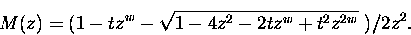(15)

Let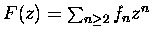. Since fn+2 = |M(n,0)|,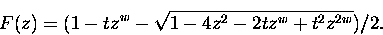(16)

Note that the coefficient of zn both in the power series for F(z) and in the power series for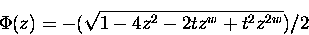must agree for all coefficients fn, except for n = 0 or n = w. Logarithmic differentiation yields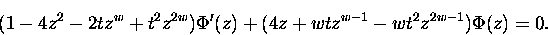Upon comparing coefficients we obtain a sequence of recurrences, where we denote the nth recurrence in the sequence as
 RECUR(n): n fn = 4(n-3) fn-2 + (2n - 3 w) t fn-w - (n - 3 w) t2 fn-2w
This recurrence is valid, yielding Proposition 2, except when the term f0 or the term fw is present. The term fw appears in four recurrences, namely, RECUR(n) for n = w, n = w + 2, n = 2w and n = 3w. In the highest indexed recurrence of these four, namely,
 RECUR(3w): 3wf3w = 4(3w-3) f3w-2 + 3wt f2w - 0 t2 fw,
there is no requirement placed on the value of fw. Hence the recurrence of (5) is valid for n > 2w. We obtain the initial conditions for (5) by enumerating the ways to insert either none or one horizontal step in each of the appropriate paths of E(n,0) for w = 0, for n <= 2 w, which are enumerated in Proposition 1.

4.   Factorial moments

Here we extend a method for summing path ordinates, given by Woan, Shapiro, and Rogers , to one for summing falling factorials of ordinates for paths of E(n,0). For a positive integer r we will consider the t-weighted sum of the falling factorial moments, given by (with the divisor n - 1 missing)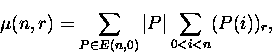where (k)r denotes the falling factorial. That is, (k)r = k(k-1)r-1 for positive integer r and (k)0 = 1. The formulation of the next proposition is based on the studies of Shapiro, Woan, and Getu  and of Chapman , both of which considered moments of all degrees for Dyck paths, with  considering rising moments.

Proposition 5.   For integer r, r >= 1,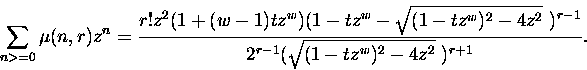Proof. We use the following temporary notation. Let B(A) = 1 if A is a true statement and B(A) = 0 if is false. Let (i,k) step end of P'' abbreviate (i,k) is an end point of a step of path P''. Let (i,k) interior of P'' abbreviate (i,k) is an interior lattice point on a horizontal step of path P''. Such a horizontal step will run from (j,k) to (j+w,k) in our notation. Let Q be any path in E(j,k). Let R and R' denote arbitrary paths that never pass below the x-axis with R running from (j,k) to (n,0) and R' running from (j+w,k) to (n,0). By symmetry, R can be matched with a path in E(n-j,k). Let m(j,k) denote |E(j,k)|.

For n >= 2,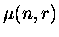=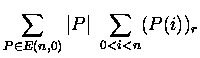=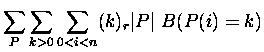=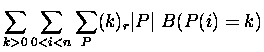=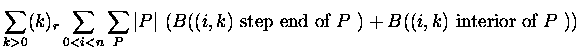=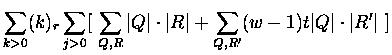=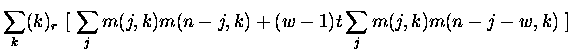With M denoting M(z), we claim that the following string of equations holds: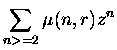=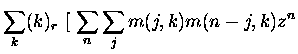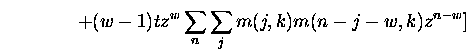=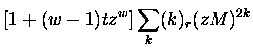(17) =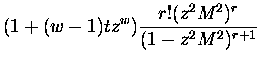(18) =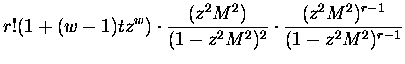(19) =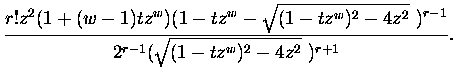To establish this string we first note that each path in E(j,k) must depart from each line y = c, for integer c, 0 <= c < k, for a last time. Hence a simple convolution argument shows that the generating function for m(j,k) satisfies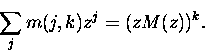(20)

This implies (17). Line (18) is a consequence of binomial theorem in the form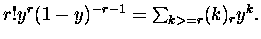To handle the middle fraction in (19) we use (14) twice: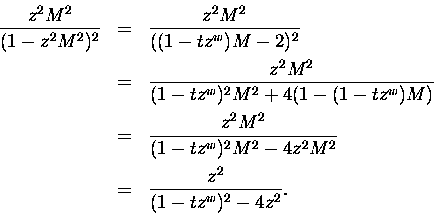To handle the last fraction in (19) use the following result derived from formula (15), with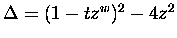: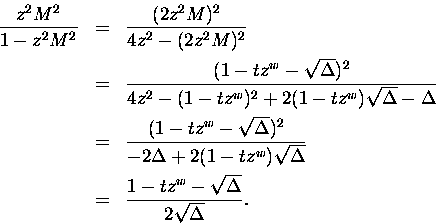5.   Area and second moments

Setting r = 1 in Proposition 5, we obtain a generating function for sums of the t-weighted areas: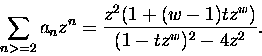(21)

Then the first part of Proposition 3 follows upon comparing coefficients in (21), rewritten as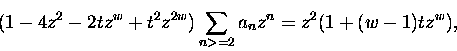and checking the obvious initial conditions. Recurrence (6) is then immediately derived from (12) by (2) and (3).

There is an interesting corollary when w = 1. Using partial fractions decomposition, the generating function (21) yields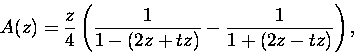and so, for w = 1 and n >= 2,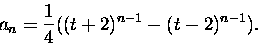To obtain the generating function for the second moments,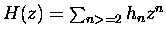, we use the following, where the constant of integration is checked to be 0:

 H(z) =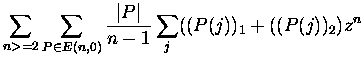=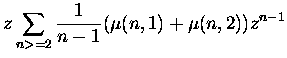=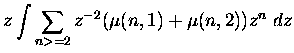=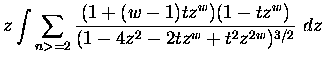=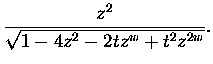(22)

Let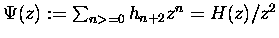. From (22), differentiation with respect to z yields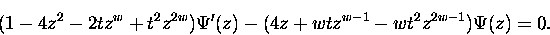Hence
( nhn+2 - 4 (n-2) hn - 2 (n-w+2)t hn-w+2 + (n-2w+2) t2 hn-2w+2 )
- ( 4 hn + wt hn-w+2 - 2 wt2 hn-2w+2 ) = 0.
Shifting the index yields the first part of Proposition 4, namely (7), where the initial conditions are easily checked.

6.   Relating second moments to the central numbers

We begin with a straightforward extension of the André reflection method to paths that contain horizontal steps. We obtain the following string of bijections:

 M(n,0) = U(n,0) - { P  in  U(n,0) :  P  intersects the line  y = -1} <-> U(n,0) - { P'  :  P' runs from  (0,-2)  to  (n,0) } (23) <-> U(n,0) -  U(n,2). (24)
To obtain (23), observe that each path   P   in the set  { P  in  U(n,0) :  P  intersects the line  y=-1}  can be decomposed as P = QR, where Q terminates at the first intercept of the line y = -1 by the path P. Let Q' denote the reflection of the path Q about the line y = -1. The matching P = QR with P' =Q'R now defines the bijection indicated in (23). A simple translation yields (24).

Let u(x,y) denote |U(x,y)|. Since any path to the point (n+1,k) must end with a U, D, or H step, we have

u(n+1,k) = u(n, k-1) + u(n, k+1) + t u(n-w+1)

and u(n, -1) = u(n, 1). Using (24) and these identities, we obtain

 2fn+2 = 2u(n,0) - 2u(n,2) = 4 u(n,0) - 2u(n+1,1) +2 t u(n-w+1,1) = 4 u(n,0) + t u(n-w+2,0)- u(n+2,0) - (t2u(n-2w+2,0)-t u(n-w+2,0)) = -u(n+2,0) + 4 u(n,0) + 2 t u(n-w+2,0) -t2 u(n-2w+2,0). (25)

Returning to results (16) and (22), we observe that they imply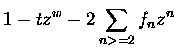=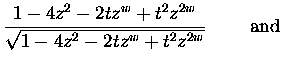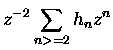=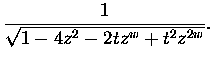Comparing coefficients yields the mixed recurrence

 2fn+2 = - hn+4 + 4 hn+2 + 2t hn-w+4 - t2 hn-2w+4. (26)

But this recurrence for fn and hn has the same form as that for fn and u(n,0) given in (25). Since the initial conditions agree, we have the second statement of Proposition 4 by induction. Moreover, we have that the generating function for u(n,0) = |U(n,0)| satisfies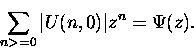We have omitted the explicit formulas for fn and hn, which are weighted sums of Catalan and binomial coefficients, respectively. In light of (13), we can find these sums by counting the ways to insert horizontal steps into the respective paths.

The first formula of (11) yields the following known relation between the Catalan numbers and the central binomial numbers. Upon replacing 2k+2 by n in that formula, we find for h = 0, n >= 2 and n even,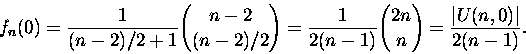The following gives an analogous result for general w:

Proposition 6.   For n > 2w,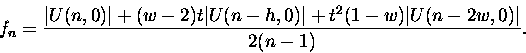Proof: One can substitute expressions given by recurrence (7) and (26) into (5), which is valid for n > 2w. Our substitutions were facilitated using a computer algebra program. Equation (13) then is applied to complete the proof.

7.   Examples

In Table 2, we record the previously studied, and named, examples satisfying the recurrences in Propositions 1 to 4, along with their reference number from Sloane's On-Line Encyclopedia of Integer Sequences . These examples correspond to sets of elevated paths, (E(n,0) )n >= 2, so in the table n = 2, 3, 4 . . . and k = 1, 2, 3 . . . .

 t Sequence Name Sloane 1 fn(0) 1, 0, 1, 0, 2, 0, 5, 0, 14, 0, 42 aerated Catalan nos. 1 f2k(0) 1, 1, 2, 5, 14, 42, 132, 429, 1430, 4862 Catalan nos. A000108 1 a2k(0) 1, 4, 16, 64, 256, 1024, 4096, 16384 powers of 4 A000302 1 h2k(0) 1, 2, 6, 20, 70, 252, 924, 3432, 12870 central binomial nos. A000984 1 fn(1 ) 1, 1, 2, 4, 9, 21, 51, 127, 323, 835, 2188 Motzkin nos. A001006 2 fn(1 ) 1, 2, 5, 14, 42, 132, 429, 1430, 4862 (lacking initial 1) A000108 3 fn(1 ) 1, 3, 10, 36, 137, 543, 2219, 9285, 39587 (tree-like polyhexes) A002212 4 fn(1 ) 1, 4, 17, 76, 354, 1704, 8421, 42508 (walks on cubic lattice) A005572 1 an(1) 1, 2, 7, 20, 61, 182, 547, 1640, 4921 A014983 1 hn(1 ) 1, 1, 3, 7, 19, 51, 141, 393, 1107, 3139 central trinomial nos. A002426 1 f2k(2) 1, 2, 6, 22, 90, 394, 1806, 8558, 41586 large Schröder A006318 1 a2k(2) 1, 7, 41, 239, 1393, 8119, 47321, 275807 A002315 1 h2k(2) 1, 3, 13, 63, 321, 1683, 8989, 48639 central Delannoy nos. A001850 1 fn(3 ) 1, 0, 1, 1, 2, 3, 6, 10, 20, 36, 72, 136 A005418 1 an(3) 1, 0, 4, 4, 16, 24, 71, 128, 328, 650 A053441 1 hn(3 ) 1, 0, 2, 1, 6, 6, 21, 30, 82, 141, 342, 650 A053442

Table 2

In Table 2, the walks on cubic lattice'' entry illustrates one role played by the indeterminate t. Corresponding to the case w = 1 and t = 4, Guy  found fn (mildly re-indexed) to count the walks on a three-dimensional lattice that use unit steps in all six standard directions (i.e. the positive and negative unit steps parallel to the three axes), that start at (0,0,0), end on the x-y plane, and never pass beneath that plane. More generally, for m > 3, w = 1 and t = 2m-1, we find that fn counts the walks of length n-2 on the m-dimensional integer lattice that use the unit steps in all 2m standard directions, start at the origin, end on the hyperplane, x1 + ... +xm-1 = 0, and never pass through a lattice point (x1, ... , xm) for which xm < 0. To see this we identify the unit step in the positive xm direction with the U-step, the unit step in the negative xm direction with the D-step, and the set of the other 2m-1 steps, none of which affects the distance from the hyperplane, x1 + ... +xm-1 = 0, with a weighed H-step.

Another example utilizing t is the enumeration of the horizontal steps over all paths in E(n,0). Let fn,k denote the number of paths in E(n,0) having k horizontal steps. We find that the generating function for the total number of horizontal steps on paths having k horizontal steps satisfies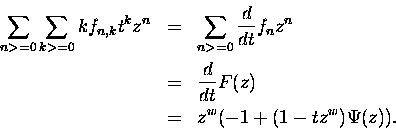Consequentially, the total number of horizontal steps is expressible in terms of t-weighted unrestricted paths as follows: for n >= 0,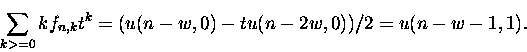8.   Related Studies

As noted in , in the 1730's, Ming An-tu, a Mongolian mathematician, was aware of the Catalan numbers, (cn)n>=0 = (1, 1, 2, 5, 14, ...), in a non-combinatorial sitting. He discovered several recurrence for these numbers including the well-known convolution recurrence, cn = c0cn-1 + c1cn-2 + . . . + cn-1c0. In about 1758, Euler  and Segner  made the first European discovery of these numbers while counting the triangulations of a convex polygon. They observed that cn-2 is the number of ways to draw non-crossing diagonals between the vertices of a convex n-gon. Segner recorded and proved the above convolution recurrence in terms of triangulations of polygons. Euler observed, without giving a proof, that cn = (4n-2)/(n+1) cn-1, which is essentially (8), and then gave a closed form for cn as a product of ratios that reduces to a ratio of product as in the first formula of (11).

There are several studies on lattice paths, in addition to those mentioned previously, that have influenced our results. Barcucci, Pinzani, and Sprugnoli  have made a systematic analysis containing recurrences - many mixed - for the Motzkin paths, i.e. w = 1 and t = 1, involving the sequences for count, central entries (central trinomial coefficients), and other related statistics. Recently the author  has established (5), (6), and (7) bijectively for Motzkin paths.

Besides Kreweras , Bonin, Shapiro, and Simion  have considered elevated Schröder paths and the recurrence (12) for w = 2. For w = 2 the author  has employed bijective schema to establish recurrences (5) and (12); in  he has considered (5), (6), and (7) in terms of parallelogram polyominoes. Most recently for w = 2, Merlini, Sprugnoli, and Verri  have given additional proofs for (5) with essentially an arbitrary t, while Pergola and Pinzani  have developed an encoding relating area to path count to obtain (12) bijectively.

Merlini, Sprugnoli, and Verri  have developed generating functions for the total area bounded by lattice paths where the permitted steps are more general than our U, D, and H. For Dyck paths, Chapman  has considered the generating functions for the sums of path moments and the relationship between the generating functions for elevated versus non-elevated paths.

Stanley  has given an extensive treatment of generalizations of the central entries, |U(n,0)|, under the name diagonals''. In  his results for differentiably finite power series relate to our use of the generating functions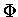and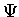of Sections 3 and 5. Correspondingly, he considered polynomially recursive sequences, for which our sequences (fn), (gn), and (hn) are prime examples.

Acknowledgements: The author thanks Lou Shapiro for sharing an early draft of  and for his suggestions improving this paper. The author also thanks Joyce Sulanke for her assistance in translating , , and .

Bibliography

1
E. Barcucci, R. Pinzani and R. Sprugnoli, The Motzkin family, Pure Math. Appl. Ser. A 2 (1992), no. 3-4, 249-279.

2
J. Bonin, L. Shapiro, and R. Simion, Some q-analogues of the Schröder numbers arising from combinatorial statistics on lattice paths, J. Statistical Planning and Inference 34 (1993) 35-55.

3
R. Chapman, Moments of Dyck paths, Disc. Math., 204 (1999), 113-117.

4
R. Donaghey and L. Shapiro, Motzkin numbers, J. of Comb. Th., ser. A 23 (1979) 291-301.

5
L. Euler, Summarium, Novi Commentarii Acad. Sci. Petropolitanae, 7 (1758-59) 13-15.

6
L. Euler, Observationes Analyticae, Novi Commentarii Acad. Sci. Petropolitanae, 11 (1765) 124 - 143.

7
R. K. Guy, Catwalks, Sandsteps and Pascal Pyramids, J. Integer Sequences, 3 (2000), to appear.

8
Luo Jian-Jin, Catalan numbers in the history of mathematics in China. Combinatorics and Graph Theory: Proc. Spring School and International Conference on Combinatorics, Hefei, H.P. Yap et al, editors, World Scientific, 1993, pp. 68-70.

9
G. Kreweras, Aires des chemins surdiagonaux a étapes obliques permises. Cahier du B.U.R.O. 24 (1976) 9-18.

10
D. Merlini, R. Sprugnoli, and M. C. Verri, The area determined by underdiagonal lattice paths, Proceedings of CAAP'96, Lecture Notes in Computer Science 1059, 59-71, 1996.

11
D. Merlini, R. Sprugnoli, and M. C. Verri, An algebraic-combinatorial approach for studying coloured Dyck-Schröder paths, preprint 1999.

12
T. Motzkin, Relations between hypersurface cross ratios, and a combinatorial formula for partitions of a polygon, for permanent preponderance, and for non-associative products, Bull. Amer. Math. Soc. 54 (1948) 352-360.

13
E. Pergola and R. Pinzani, A Combinatorial Interpretation of the Area of Schröder Paths, preprint, 1999.

14
A. de Segner, Enumeratio modorum, quibus figurae planae rectilineae per diagonales dividuntur in triangula, Novi Commentarii Acad. Sci. Petropolitanae, 7 (1758-59) 203-209.

15
L. Shapiro, W-J Woan, and S. Getu, Runs, slides, and moments, SIAM, J. of Disc. Math. 4, (1983) 459-466.

16
N. J. A. Sloane, The On-Line Encyclopedia of Integer Sequences, published electronically at www.research.att.com/~njas/sequences/.

17
R. P. Stanley, Enumerative Combinatorics, Vol. 2, Cambridge University Press, 1999

18
R. A. Sulanke, Bijective Recurrences concerning Schröder Paths, Electronic Journal of Combinatorics, Vol. 5(1), R47, 1998

19
R. A. Sulanke, Three Recurrences for Parallelogram Polyominoes, J. of Difference Eq. and its Appl., 5 (1999) 155-176.

20
R. A. Sulanke, Bijective Recurrences for Motzkin Paths, in preparation, 1999.

21
W-J Woan, L. Shapiro, and D. G. Rogers, The Catalan numbers, the Lebesgue integral and 4n-2, Am. Math. Monthly, 104, (1997) 926-931.

(This paper uses lattice paths to produce a unified treatment of sequences A000108, A000302, A000984, A001006, A001850, A002212, A002315, A002426, A005418, A005572, A006318, A014983, A053441, A053442 in the On-Line Encyclopedia of Integer Sequences.)

Received Nov. 11 1999. Published in Journal of Integer Sequences Jan. 12, 2000.